# Transformer Basics and Transformer Principles

Home Forums Engineering Electrical / Electronic Engineers Transformer Basics and Transformer Principles

• Author
Posts
• #39618

# Transformer Basics

Transformers are electrical devices consisting of two or more coils of wire used to transfer electrical energy by means of a changing magnetic field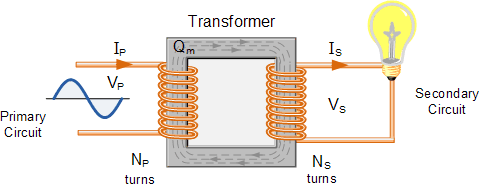One of the main reasons that we use alternating AC voltages and currents in our homes and workplace’s is that AC supplies can be easily generated at a convenient voltage, transformed (hence the name transformer) into much higher voltages and then distributed around the country using a national grid of pylons and cables over very long distances.

The reason for transforming the voltage to a much higher level is that higher distribution voltages implies lower currents for the same power and therefore lower I2*R losses along the networked grid of cables. These higher AC transmission voltages and currents can then be reduced to a much lower, safer and usable voltage level where it can be used to supply electrical equipment in our homes and workplaces, and all this is possible thanks to the basic Voltage Transformer.A Typical Voltage Transformer

The Voltage Transformer can be thought of as an electrical component rather than an electronic component. A transformer basically is very simple static (or stationary) electro-magnetic passive electrical device that works on the principle of Faraday’s law of induction by converting electrical energy from one value to another.

The transformer does this by linking together two or more electrical circuits using a common oscillating magnetic circuit which is produced by the transformer itself. A transformer operates on the principals of “electromagnetic induction”, in the form of  Mutual Induction.

Mutual induction is the process by which a coil of wire magnetically induces a voltage into another coil located in close proximity to it. Then we can say that transformers work in the “magnetic domain”, and transformers get their name from the fact that they “transform” one voltage or current level into another.

Transformers are capable of either increasing or decreasing the voltage and current levels of their supply, without modifying its frequency, or the amount of electrical power being transferred from one winding to another via the magnetic circuit.

A single phase voltage transformer basically consists of two electrical coils of wire, one called the “Primary Winding” and another called the “Secondary Winding”. For this tutorial we will define the “primary” side of the transformer as the side that usually takes power, and the “secondary” as the side that usually delivers power. In a single-phase voltage transformer the primary is usually the side with the higher voltage.

These two coils are not in electrical contact with each other but are instead wrapped together around a common closed magnetic iron circuit called the “core”. This soft iron core is not solid but made up of individual laminations connected together to help reduce the core’s losses.

The two coil windings are electrically isolated from each other but are magnetically linked through the common core allowing electrical power to be transferred from one coil to the other. When an electric current passed through the primary winding, a magnetic field is developed which induces a voltage into the secondary winding as shown.

### Single Phase Voltage Transformer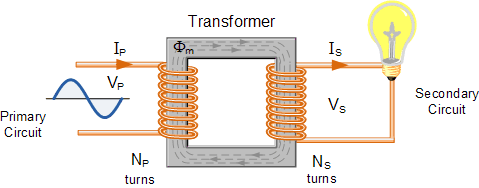In other words, for a transformer there is no direct electrical connection between the two coil windings, thereby giving it the name also of an Isolation Transformer. Generally, the primary winding of a transformer is connected to the input voltage supply and converts or transforms the electrical power into a magnetic field. While the job of the secondary winding is to convert this alternating magnetic field into electrical power producing the required output voltage as shown.

### Transformer Construction (single-phase)• Where:
•   VP  –  is the Primary Voltage
•   VS  –  is the Secondary Voltage
•   NP  –  is the Number of Primary Windings
•   NS  –  is the Number of Secondary Windings
•   Φ (phi)  –  is the Flux Linkage

Notice that the two coil windings are not electrically connected but are only linked magnetically. A single-phase transformer can operate to either increase or decrease the voltage applied to the primary winding. When a transformer is used to “increase” the voltage on its secondary winding with respect to the primary, it is called a Step-up transformer. When it is used to “decrease” the voltage on the secondary winding with respect to the primary it is called a Step-down transformer.

However, a third condition exists in which a transformer produces the same voltage on its secondary as is applied to its primary winding. In other words, its output is identical with respect to voltage, current and power transferred. This type of transformer is called an “Impedance Transformer” and is mainly used for impedance matching or the isolation of adjoining electrical circuits.

The difference in voltage between the primary and the secondary windings is achieved by changing the number of coil turns in the primary winding ( NP ) compared to the number of coil turns on the secondary winding ( NS ).

As the transformer is basically a linear device, a ratio now exists between the number of turns of the primary coil divided by the number of turns of the secondary coil. This ratio, called the ratio of transformation, more commonly known as a transformers “turns ratio”, ( TR ). This turns ratio value dictates the operation of the transformer and the corresponding voltage available on the secondary winding.

It is necessary to know the ratio of the number of turns of wire on the primary winding compared to the secondary winding. The turns ratio, which has no units, compares the two windings in order and is written with a colon, such as 3:1 (3-to-1). This means in this example, that if there are 3 volts on the primary winding there will be 1 volt on the secondary winding, 3 volts-to-1 volt. Then we can see that if the ratio between the number of turns changes the resulting voltages must also change by the same ratio, and this is true.

Transformers are all about “ratios”. The ratio of the primary to the secondary, the ratio of the input to the output, and the turns ratio of any given transformer will be the same as its voltage ratio. In other words for a transformer: “turns ratio = voltage ratio”. The actual number of turns of wire on any winding is generally not important, just the turns ratio and this relationship is given as:

### A Transformers Turns RatioAssuming an ideal transformer and the phase angles:  ΦP ≡ ΦS

Note that the order of the numbers when expressing a transformers turns ratio value is very important as the turns ratio 3:1 expresses a very different transformer relationship and output voltage than one in which the turns ratio is given as: 1:3.

## Transformer Basics Example No1

A voltage transformer has 1500 turns of wire on its primary coil and 500 turns of wire for its secondary coil. What will be the turns ratio (TR) of the transformer.This ratio of 3:1 (3-to-1) simply means that there are three primary windings for every one secondary winding. As the ratio moves from a larger number on the left to a smaller number on the right, the primary voltage is therefore stepped down in value as shown.

## Transformer Basics Example No2

If 240 volts rms is applied to the primary winding of the same transformer above, what will be the resulting secondary no load voltage.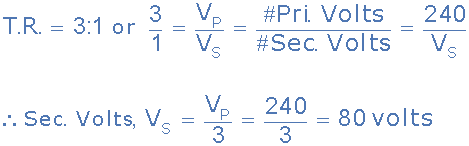Again confirming that the transformer is a “step-down” transformer as the primary voltage is 240 volts and the corresponding secondary voltage is lower at 80 volts.

Then the main purpose of a transformer is to transform voltages at preset ratios and we can see that the primary winding has a set amount or number of windings (coils of wire) on it to suit the input voltage. If the secondary output voltage is to be the same value as the input voltage on the primary winding, then the same number of coil turns must be wound onto the secondary core as there are on the primary core giving an even turns ratio of 1:1 (1-to-1). In other words, one coil turn on the secondary to one coil turn on the primary.

If the output secondary voltage is to be greater or higher than the input voltage, (step-up transformer) then there must be more turns on the secondary giving a turns ratio of 1:N (1-to-N), where N represents the turns ratio number. Likewise, if it is required that the secondary voltage is to be lower or less than the primary, (step-down transformer) then the number of secondary windings must be less giving a turns ratio of N:1 (N-to-1).

## Transformer Action

We have seen that the number of coil turns on the secondary winding compared to the primary winding, the turns ratio, affects the amount of voltage available from the secondary coil. But if the two windings are electrically isolated from each other, how is this secondary voltage produced?

We have said previously that a transformer basically consists of two coils wound around a common soft iron core. When an alternating voltage ( VP ) is applied to the primary coil, current flows through the coil which in turn sets up a magnetic field around itself, called mutual inductance, by this current flow according to Faraday’s Law of electromagnetic induction. The strength of the magnetic field builds up as the current flow rises from zero to its maximum value which is given as dΦ/dt.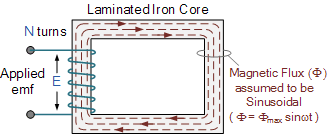As the magnetic lines of force setup by this electromagnet expand outward from the coil the soft iron core forms a path for and concentrates the magnetic flux. This magnetic flux links the turns of both windings as it increases and decreases in opposite directions under the influence of the AC supply.

However, the strength of the magnetic field induced into the soft iron core depends upon the amount of current and the number of turns in the winding. When current is reduced, the magnetic field strength reduces.

When the magnetic lines of flux flow around the core, they pass through the turns of the secondary winding, causing a voltage to be induced into the secondary coil. The amount of voltage induced will be determined by: N*dΦ/dt (Faraday’s Law), where N is the number of coil turns. Also this induced voltage has the same frequency as the primary winding voltage.

Then we can see that the same voltage is induced in each coil turn of both windings because the same magnetic flux links the turns of both the windings together. As a result, the total induced voltage in each winding is directly proportional to the number of turns in that winding. However, the peak amplitude of the output voltage available on the secondary winding will be reduced if the magnetic losses of the core are high.

If we want the primary coil to produce a stronger magnetic field to overcome the cores magnetic losses, we can either send a larger current through the coil, or keep the same current flowing, and instead increase the number of coil turns ( NP ) of the winding. The product of amperes times turns is called the “ampere-turns”, which determines the magnetising force of the coil.

So assuming we have a transformer with a single turn in the primary, and only one turn in the secondary. If one volt is applied to the one turn of the primary coil, assuming no losses, enough current must flow and enough magnetic flux generated to induce one volt in the single turn of the secondary. That is, each winding supports the same number of volts per turn.

As the magnetic flux varies sinusoidally, Φ = Φmax sinωt, then the basic relationship between induced emf, ( E ) in a coil winding of N turns is given by:

### emf = turns x rate of change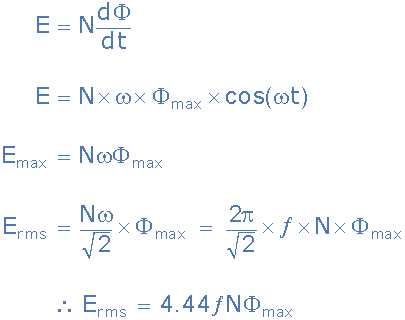• Where:
•   ƒ  –  is the flux frequency in Hertz,  = ω/2π
•   Ν  –  is the number of coil windings.
•   Φ  –  is the amount of flux in webers

This is known as the Transformer EMF Equation. For the primary winding emf, N will be the number of primary turns, ( NP ) and for the secondary winding emf, N will be the number of secondary turns, ( NS ).

Also please note that as transformers require an alternating magnetic flux to operate correctly, transformers cannot therefore be used to transform or supply DC voltages or currents, since the magnetic field must be changing to induce a voltage in the secondary winding. In other words, transformers DO NOT operate on steady state DC voltages, only alternating or pulsating voltages.

If a transformers primary winding was connected to a DC supply, the inductive reactance of the winding would be zero as DC has no frequency, so the effective impedance of the winding will therefore be very low and equal only to the resistance of the copper used. Thus the winding will draw a very high current from the DC supply causing it to overheat and eventually burn out, because as we know I = V/R.

## Transformer Basics Example No3

A single phase transformer has 480 turns on the primary winding and 90 turns on the secondary winding. The maximum value of the magnetic flux density is 1.1T when 2200 volts, 50Hz is applied to the transformer primary winding. Calculate:

a). The maximum flux in the core.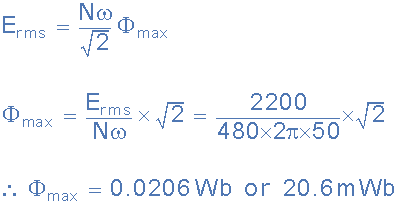b). The cross-sectional area of the core.c). The secondary induced emf.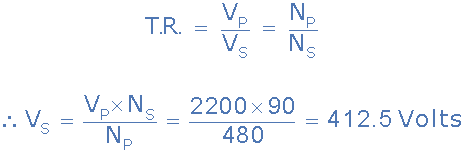## Electrical Power in a Transformer

Another one of the transformer basics parameters is its power rating. The power rating of a transformer is obtained by simply multiplying the current by the voltage to obtain a rating in Volt-amperes, ( VA ). Small single phase transformers may be rated in volt-amperes only, but much larger power transformers are rated in units of Kilo volt-amperes, ( kVA ) where 1 kilo volt-ampere is equal to 1,000 volt-amperes, and units of Mega volt-amperes, ( MVA ) where 1 mega volt-ampere is equal to 1 million volt-amperes.

In an ideal transformer (ignoring any losses), the power available in the secondary winding will be the same as the power in the primary winding, they are constant wattage devices and do not change the power only the voltage to current ratio. Thus, in an ideal transformer the Power Ratio is equal to one (unity) as the voltage, V multiplied by the current, I will remain constant.

That is the electric power at one voltage/current level on the primary is “transformed” into electric power, at the same frequency, to the same voltage/current level on the secondary side. Although the transformer can step-up (or step-down) voltage, it cannot step-up power. Thus, when a transformer steps-up a voltage, it steps-down the current and vice-versa, so that the output power is always at the same value as the input power. Then we can say that primary power equals secondary power, ( PP = PS ).

### Power in a Transformer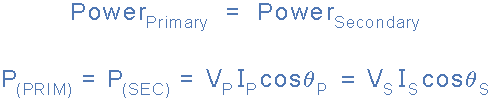Where: ΦP is the primary phase angle and ΦS is the secondary phase angle.

Note that since power loss is proportional to the square of the current being transmitted, that is: I2R, increasing the voltage, let’s say doubling ( ×2 ) the voltage would decrease the current by the same amount, ( ÷2 ) while delivering the same amount of power to the load and therefore reducing losses by factor of 4. If the voltage was increased by a factor of 10, the current would decrease by the same factor reducing overall losses by factor of 100.

## Transformer Basics – Efficiency

A transformer does not require any moving parts to transfer energy. This means that there are no friction or windage losses associated with other electrical machines. However, transformers do suffer from other types of losses called “copper losses” and “iron losses” but generally these are quite small.

Copper losses, also known as I2R loss is the electrical power which is lost in heat as a result of circulating the currents around the transformers copper windings, hence the name. Copper losses represents the greatest loss in the operation of a transformer. The actual watts of power lost can be determined (in each winding) by squaring the amperes and multiplying by the resistance in ohms of the winding (I2R).

Iron losses, also known as hysteresis is the lagging of the magnetic molecules within the core, in response to the alternating magnetic flux. This lagging (or out-of-phase) condition is due to the fact that it requires power to reverse magnetic molecules; they do not reverse until the flux has attained sufficient force to reverse them.

Their reversal results in friction, and friction produces heat in the core which is a form of power loss. Hysteresis within the transformer can be reduced by making the core from special steel alloys.

The intensity of power loss in a transformer determines its efficiency. The efficiency of a transformer is reflected in power (wattage) loss between the primary (input) and secondary (output) windings. Then the resulting efficiency of a transformer is equal to the ratio of the power output of the secondary winding, PS to the power input of the primary winding, PP and is therefore high.

An ideal transformer is 100% efficient because it delivers all the energy it receives. Real transformers on the other hand are not 100% efficient and at full load, the efficiency of a transformer is between 94% to 96% which is quiet good. For a transformer operating with a constant voltage and frequency with a very high capacity, the efficiency may be as high as 98%. The efficiency, η of a transformer is given as:

### Transformer EfficiencyWhere: Input, Output and Losses are all expressed in units of power.

Generally when dealing with transformers, the primary watts are called “volt-amps”, VA to differentiate them from the secondary watts. Then the efficiency equation above can be modified to: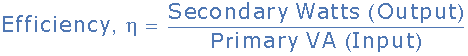It is sometimes easier to remember the relationship between the transformers input, output and efficiency by using pictures. Here the three quantities of VAW and η have been superimposed into a triangle giving power in watts at the top with volt-amps and efficiency at the bottom. This arrangement represents the actual position of each quantity in the efficiency formulas.

### Transformer Efficiency Triangle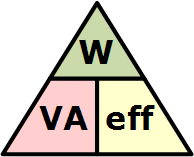and transposing the above triangle quantities gives us the following combinations of the same equation: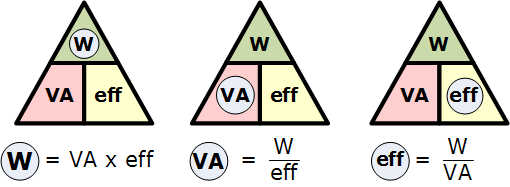Then, to find Watts (output) = VA x eff., or to find VA (input) = W/eff., or to find Efficiency, eff. = W/VA, etc.

## Transformer Basics Summary

Then to summarise this transformer basics tutorial. A Transformer changes the voltage level (or current level) on its input winding to another value on its output winding using a magnetic field. A transformer consists of two electrically isolated coils and operates on Faraday’s principal of “mutual induction”, in which an EMF is induced in the transformers secondary coil by the magnetic flux generated by the voltages and currents flowing in the primary coil winding.

Both the primary and secondary coil windings are wrapped around a common soft iron core made of individual laminations to reduce eddy current and power losses. The primary winding of the transformer is connected to the AC power source which must be sinusoidal in nature, while the secondary winding supplies electrical power to the load. Having said that, a transformer could be used in reverse with the supply connected to the secondary winding provided the voltage and current ratings are observed.

We can represent the transformer in block diagram form as follows:

### Basic Representation of the Transformer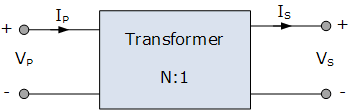The ratio of the transformers primary and secondary windings with respect to each other produces either a step-up voltage transformer or a step-down voltage transformer with the ratio between the number of primary turns to the number of secondary turns being called the “turns ratio” or “transformer ratio”.

If this ratio is less than unity, n < 1 then NS is greater than NP and the transformer is classed as a step-up transformer. If this ratio is greater than unity, n > 1, that is NP is greater than NS, the transformer is classed as a step-down transformer. Note that single phase step-down transformer can also be used as a step-up transformer simply by reversing its connections and making the low voltage winding its primary, and vice versa as long as the transformer is operated within its original VA design rating.

If the turns ratio is equal to unity, n = 1 then both the primary and secondary have the same number of windings, therefore the voltages and currents are the same for both windings.

This type of transformer is classed as an isolation transformer as both the primary and secondary windings of the transformer have the same number of volts per turn. The efficiency of a transformer is the ratio of the power it delivers to the load to the power it absorbs from the supply. In an ideal transformer there are no losses so no loss of power then PIN = POUT.